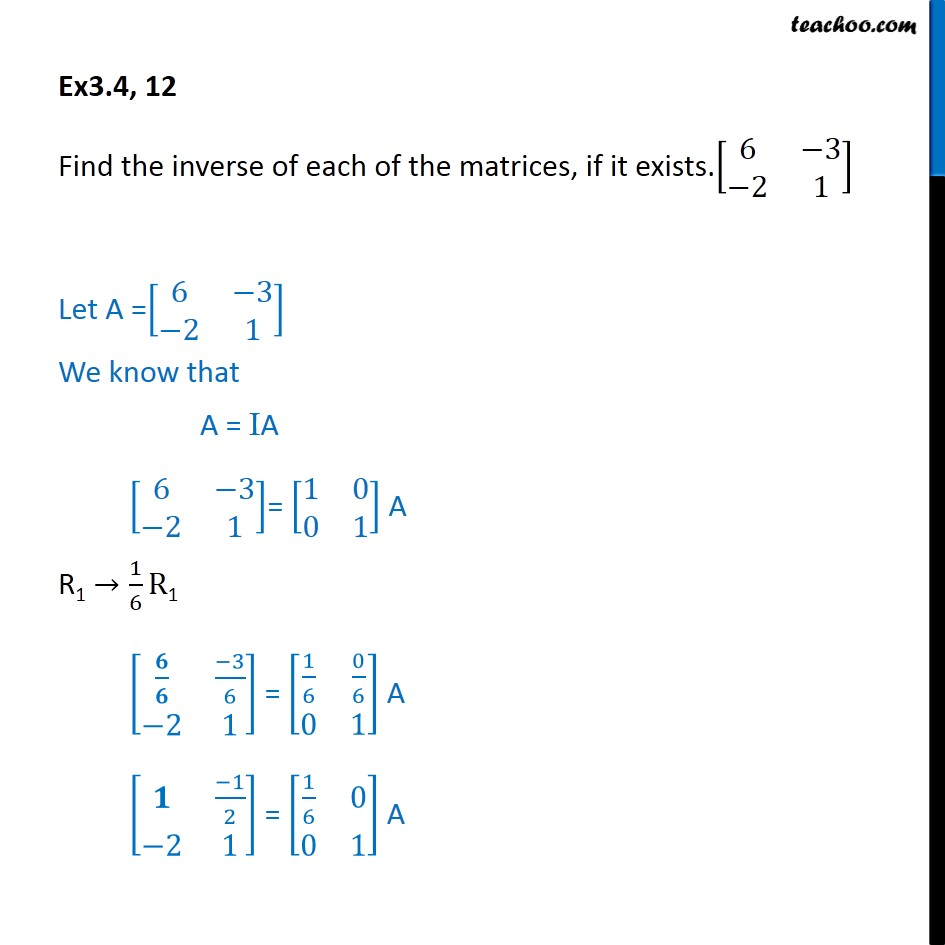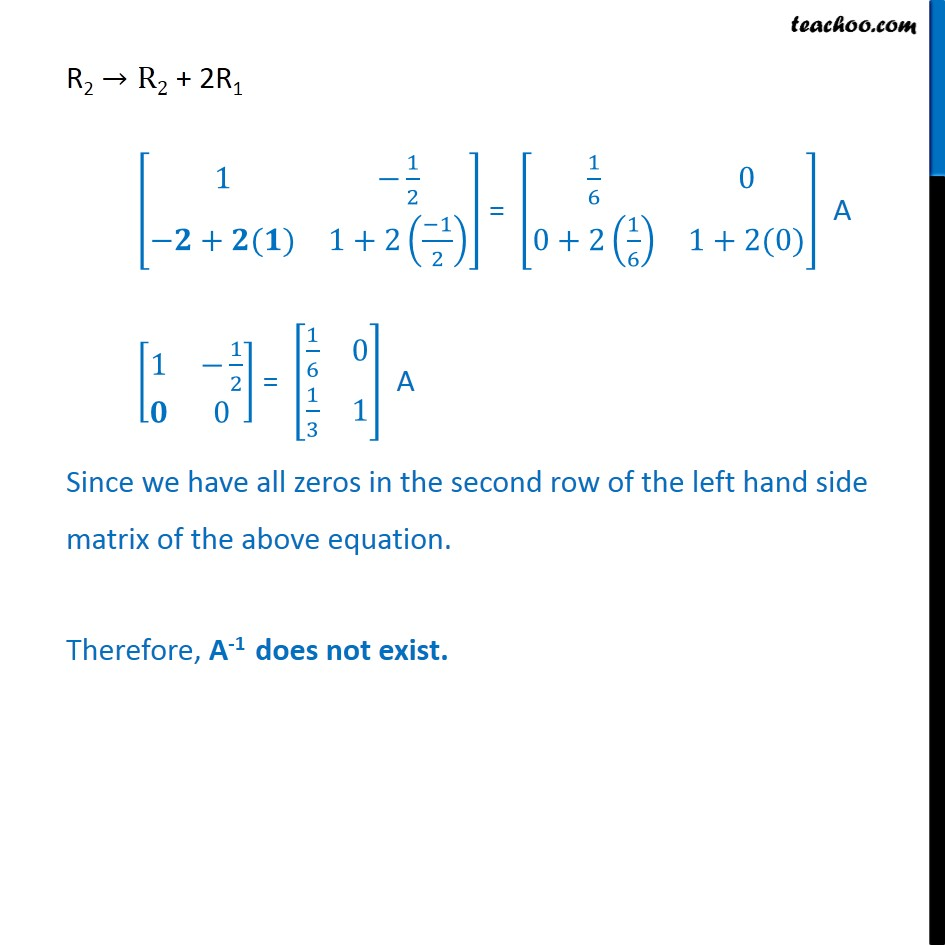1. Chapter 3 Class 12 Matrices
2. Concept wise
3. Inverse of matrix using elementary transformation

Transcript

Ex3.4, 12 Find the inverse of each of the matrices, if it exists.[■8(6&−3@−2&1)] Let A =[■8(6&−3@−2&1)] We know that A = IA [■8(6&−3@−2&1)]= [■8(1&0@0&1)] A R1 →1/6 R1 [■8(𝟔/𝟔&(−3)/6@−2&1)] = [■8(1/6&0/6@0&1)] A [■8(𝟏&(−1)/2@−2&1)] = [■8(1/6&0@0&1)] A R2 →R2 + 2R1 [■8(1&−1/2@−𝟐+𝟐(𝟏)&1+2((−1)/2) )] = [■8(1/6&0@0+2(1/6)&1+2(0))] A [■8(1&−1/2@𝟎&0)] = [■8(1/6&0@1/3&1)] A Since we have all zeros in the second row of the left hand side matrix of the above equation. Therefore, A-1 does not exist.

Inverse of matrix using elementary transformation